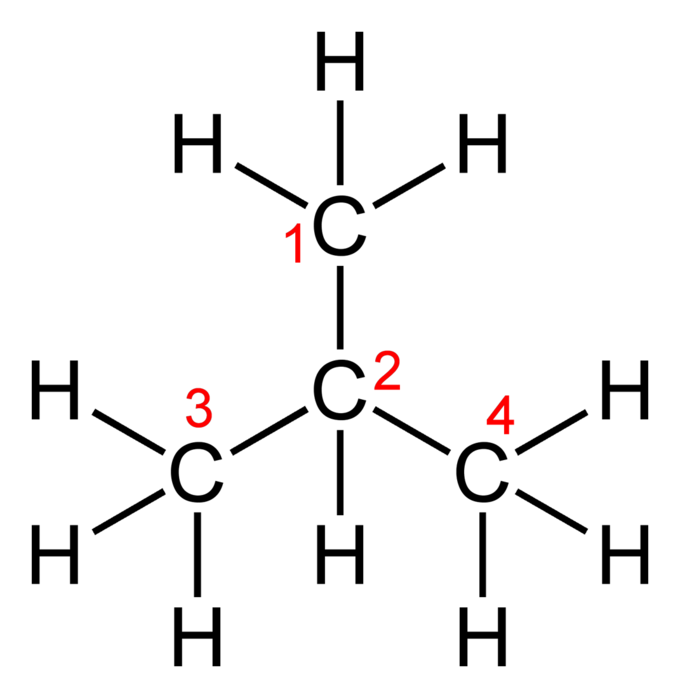## Empirical Formulas

#### Learning Objective

• Derive a molecule’s empirical formula given its mass composition

#### Key Points

• Empirical formulas are the simplest form of notation.
• The molecular formula for a compound is equal to, or a whole-number multiple of, its empirical formula.
• Like molecular formulas, empirical formulas are not unique and can describe a number of different chemical structures or isomers.
• To determine an empirical formula, the mass composition of its elements can be used to mathematically determine their ratio.

#### Term

• empirical formulaA notation indicating the ratios of the various elements present in a compound, without regard to the actual numbers.

Chemists use a variety of notations to describe and summarize the atomic constituents of compounds. These notations, which include empirical, molecular, and structural formulas, use the chemical symbols for the elements along with numeric values to describe atomic composition.

Empirical formulas are the simplest form of notation. They provide the lowest whole-number ratio between the elements in a compound. Unlike molecular formulas, they do not provide information about the absolute number of atoms in a single molecule of a compound. The molecular formula for a compound is equal to, or a whole-number multiple of, its empirical formula.

## Structural Formulas v. Empirical Formulas

An empirical formula (like a molecular formula) lacks any structural information about the positioning or bonding of atoms in a molecule. It can therefore describe a number of different structures, or isomers, with varying physical properties. For butane and isobutane, the empirical formula for both molecules is C2H5, and they share the same molecular formula, C4H10. However, one structural representation for butane is CH3CH2CH2CH3, while isobutane can be described using the structural formula (CH3)3CH.ButaneThe structural formula of butane.IsobutaneThe structural formula of isobutane.

## Determining Empirical Formulas

Empirical formulas can be determined using mass composition data. For example, combustion analysis can be used in the following manner:

• A CHN analyzer (an instrument that can determine the composition of a molecule) can be used to find the mass fractions of carbon, hydrogen, oxygen, and other atoms for a sample of an unknown organic compound.
• Once the relative mass contributions of elements are known, this information can be converted into moles.
• The empirical formula is the lowest possible whole-number ratio of the elements.

## Example 1:

Suppose you are given a compound such as methyl acetate, a solvent commonly used in paints, inks, and adhesives. When methyl acetate was chemically analyzed, it was discovered to have 48.64% carbon (C), 8.16% hydrogen (H), and 43.20% oxygen (O). For the purposes of determining empirical formulas, we assume that we have 100 g of the compound. If this is the case, the percentages will be equal to the mass of each element in grams.

Step 1: Change each percentage to an expression of the mass of each element in grams. That is, 48.64% C becomes 48.64 g C, 8.16% H becomes 8.16 g H, and 43.20% O becomes 43.20 g O because we assume we have 100 g of the overall compound.

Step 2: Convert the amount of each element in grams to its amount in moles.

$\left(\frac{48.64 \mbox{ g C}}{1}\right)\left(\frac{1 \mbox{ mol }}{12.01 \mbox{ g C}}\right) = 4.049\ \text{mol}$

$\left(\frac{8.16 \mbox{ g H}}{1}\right)\left(\frac{1 \mbox{ mol }}{1.008 \mbox{ g H}}\right) = 8.095\ \text{mol}$

$\left(\frac{43.20 \mbox{ g O}}{1}\right)\left(\frac{1 \mbox{ mol }}{16.00 \mbox{ g O}}\right) = 2.7\ \text{mol}$

Step 3: Divide each of the mole values by the smallest of the mole values.

$\frac{4.049 \mbox{ mol }}{2.7 \mbox{ mol }} = 1.5$

$\frac{8.095 \mbox{ mol }}{2.7 \mbox{ mol }} = 3$

$\frac{2.7 \mbox{ mol }}{2.7 \mbox{ mol }} = 1$

Step 4: If necessary, multiply these numbers by integers in order to get whole numbers; if an operation is done to one of the numbers, it must be done to all of them.

$1.5 \times 2 = 3$

$3 \times 2 = 6$

$1 \times 2 = 2$

Thus, the empirical formula of methyl acetate is C3H6O2.

## Example 2:

The empirical formula of decane is C5H11. Its molecular weight is 142.286 g/mol. What is the molecular formula of decane?

Step 1: Calculate the molecular weight of the empirical formula (the molecular weight of C = 12.011 g/mol and H = 1.008 g/mol)

5 (12.0111 g/mol) + 11 (1.008 g/mol) = C5H11

60.055 g/mol + 11.008 g/mol = 71.143 g/mol per C5H11

Step 2: Divide the molecular weight of the molecular formula by the the molecular weight of the empirical formula to find the ratio between the two.

$\frac{142.286 \ g/mol}{71.143 \ g/mol} = 2$

Since the weight of the molecular formula is twice the weight of the empirical formula, there must be twice as many atoms, but in the same ratio. Therefore, if the empirical formula of decane is C5H11, the molecular formula of decane is twice that, or C10H22.